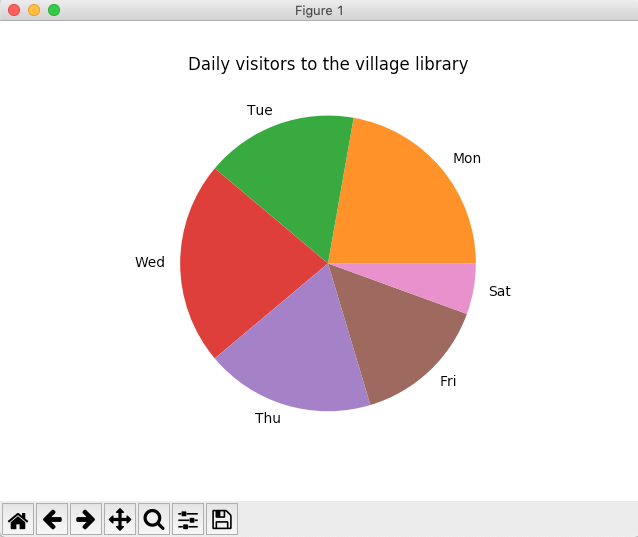# Drawing A Pie Chart Using A Pandas Series

## Overview:

• Pie charts display how much a specific variable or quantity contributes to the whole, where the whole represents 100%.
• Each variable is represented as a wedge.
• The data with a value zero will not have any wedge in the pie chart.
• Calling the pie() function of the plot member on a pandas Series instance, plots the pie chart for the Series data.

## Example:

 # Example Python Program to draw a pie chart for the data # present in a pandas Series object import pandas as pds import matplotlib.pyplot as plt   # Daily visitors data = [0,120,90,120,100,80,30];   # Create a pandas series series = pds.Series(data, index=("Sun", "Mon", "Tue", "Wed", "Thu", "Fri", "Sat"));   # Create a pie chart series.plot.pie(label="", title="Daily visitors to the village library");   plt.show(block=True);

## Output: